Search by Topic

Resources tagged with Squares similar to Treasure Island:

Filter by: Content type:
Age range:
Challenge level:

There are 50 results

Broad Topics > Angles, Polygons, and Geometrical Proof > SquaresEight Hidden Squares

Age 7 to 14 Challenge Level:

On the graph there are 28 marked points. These points all mark the vertices (corners) of eight hidden squares. Can you find the eight hidden squares?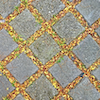Seeing Squares

Age 5 to 11 Challenge Level:

Players take it in turns to choose a dot on the grid. The winner is the first to have four dots that can be joined to form a square.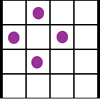Square Corners

Age 7 to 11 Challenge Level:

What is the greatest number of counters you can place on the grid below without four of them lying at the corners of a square?Square Coordinates

Age 11 to 14 Challenge Level:

A tilted square is a square with no horizontal sides. Can you devise a general instruction for the construction of a square when you are given just one of its sides?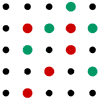Seeing Squares for Two

Age 5 to 11 Challenge Level:

Seeing Squares game for an adult and child. Can you come up with a way of always winning this game?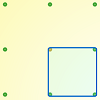Peg and Pin Boards

Age 5 to 11

This article for teachers suggests activities based on pegboards, from pattern generation to finding all possible triangles, for example.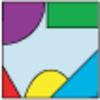Jig Shapes

Age 5 to 11 Challenge Level:

Can you each work out what shape you have part of on your card? What will the rest of it look like?Baravelle

Age 7 to 16 Challenge Level:

What can you see? What do you notice? What questions can you ask?Hidden Squares

Age 11 to 14 Challenge Level:

Can you find the squares hidden on these coordinate grids?Cut and Make

Age 7 to 11 Challenge Level:

Cut a square of paper into three pieces as shown. Now,can you use the 3 pieces to make a large triangle, a parallelogram and the square again?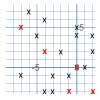Ten Hidden Squares

Age 7 to 11 Challenge Level:

These points all mark the vertices (corners) of ten hidden squares. Can you find the 10 hidden squares?Square It

Age 11 to 16 Challenge Level:

Players take it in turns to choose a dot on the grid. The winner is the first to have four dots that can be joined to form a square.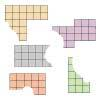Torn Shapes

Age 7 to 11 Challenge Level:

These rectangles have been torn. How many squares did each one have inside it before it was ripped?Inside Seven Squares

Age 7 to 11 Challenge Level:

What is the total area of the four outside triangles which are outlined in red in this arrangement of squares inside each other?Zooming in on the Squares

Age 7 to 14

Start with a large square, join the midpoints of its sides, you'll see four right angled triangles. Remove these triangles, a second square is left. Repeat the operation. What happens?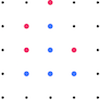Square Surprise

Age 5 to 11 Challenge Level:

Why do you think that the red player chose that particular dot in this game of Seeing Squares?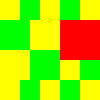Tiles on a Patio

Age 7 to 11 Challenge Level:

How many ways can you find of tiling the square patio, using square tiles of different sizes?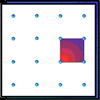Geoboards

Age 7 to 11 Challenge Level:

This practical challenge invites you to investigate the different squares you can make on a square geoboard or pegboard.Rope Mat

Age 7 to 11 Challenge Level:

How many centimetres of rope will I need to make another mat just like the one I have here?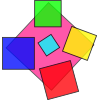Making Squares

Age 7 to 11

Investigate all the different squares you can make on this 5 by 5 grid by making your starting side go from the bottom left hand point. Can you find out the areas of all these squares?Tetra Square

Age 11 to 14 Challenge Level:

ABCD is a regular tetrahedron and the points P, Q, R and S are the midpoints of the edges AB, BD, CD and CA. Prove that PQRS is a square.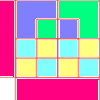Diagrams

Age 7 to 11 Challenge Level:

A group activity using visualisation of squares and triangles.Coloured Edges

Age 11 to 14 Challenge Level:

The whole set of tiles is used to make a square. This has a green and blue border. There are no green or blue tiles anywhere in the square except on this border. How many tiles are there in the set?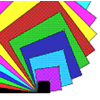Overlapping Squares

Age 7 to 11 Challenge Level:

Have a good look at these images. Can you describe what is happening? There are plenty more images like this on NRICH's Exploring Squares CD.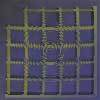My New Patio

Age 7 to 11 Challenge Level:

What is the smallest number of tiles needed to tile this patio? Can you investigate patios of different sizes?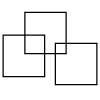Three Squares

Age 5 to 11 Challenge Level:

What is the greatest number of squares you can make by overlapping three squares?Dissect

Age 11 to 14 Challenge Level:

What is the minimum number of squares a 13 by 13 square can be dissected into?Fitted

Age 7 to 11 Challenge Level:

Nine squares with side lengths 1, 4, 7, 8, 9, 10, 14, 15, and 18 cm can be fitted together to form a rectangle. What are the dimensions of the rectangle?Squares in Rectangles

Age 11 to 14 Challenge Level:

A 2 by 3 rectangle contains 8 squares and a 3 by 4 rectangle contains 20 squares. What size rectangle(s) contain(s) exactly 100 squares? Can you find them all?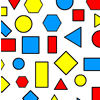Sorting Logic Blocks

Age 5 to 11 Challenge Level:

This interactivity allows you to sort logic blocks by dragging their images.2001 Spatial Oddity

Age 11 to 14 Challenge Level:

With one cut a piece of card 16 cm by 9 cm can be made into two pieces which can be rearranged to form a square 12 cm by 12 cm. Explain how this can be done.Chess

Age 11 to 14 Challenge Level:

What would be the smallest number of moves needed to move a Knight from a chess set from one corner to the opposite corner of a 99 by 99 square board?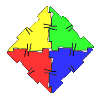Polydron

Age 7 to 11 Challenge Level:

This activity investigates how you might make squares and pentominoes from Polydron.Square Within a Square Within...

Age 11 to 14 Short Challenge Level:

What fraction of this square is shaded?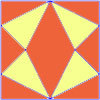Fraction Fascination

Age 7 to 11 Challenge Level:

This problem challenges you to work out what fraction of the whole area of these pictures is taken up by various shapes.On the Edge

Age 11 to 14 Challenge Level:

If you move the tiles around, can you make squares with different coloured edges?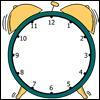A Square in a Circle

Age 7 to 11 Challenge Level:

What shape has Harry drawn on this clock face? Can you find its area? What is the largest number of square tiles that could cover this area?Squares, Squares and More Squares

Age 11 to 14 Challenge Level:

Can you dissect a square into: 4, 7, 10, 13... other squares? 6, 9, 12, 15... other squares? 8, 11, 14... other squares?Transformations on a Pegboard

Age 7 to 11 Challenge Level:

How would you move the bands on the pegboard to alter these shapes?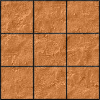Paving the Way

Age 11 to 14 Challenge Level:

A man paved a square courtyard and then decided that it was too small. He took up the tiles, bought 100 more and used them to pave another square courtyard. How many tiles did he use altogether?Square Areas

Age 11 to 14 Challenge Level:

Can you work out the area of the inner square and give an explanation of how you did it?LOGO Challenge 5 - Patch

Age 11 to 16 Challenge Level:

Using LOGO, can you construct elegant procedures that will draw this family of 'floor coverings'?Opposite Vertices

Age 11 to 14 Challenge Level:

Can you recreate squares and rhombuses if you are only given a side or a diagonal?LOGO Challenge - the Logic of LOGO

Age 11 to 16 Challenge Level:

Just four procedures were used to produce a design. How was it done? Can you be systematic and elegant so that someone can follow your logic?Great Squares

Age 7 to 11 Challenge Level:

Investigate how this pattern of squares continues. You could measure lengths, areas and angles.LOGO Challenge 7 - More Stars and Squares

Age 11 to 16 Challenge Level:

Can you use LOGO to create a systematic reproduction of a basic design? An introduction to variables in a familiar setting.LOGO Challenge 4 - Squares to Procedures

Age 11 to 16 Challenge Level:

This LOGO Challenge emphasises the idea of breaking down a problem into smaller manageable parts. Working on squares and angles.Logo Challenge 3 - Star Square

Age 7 to 16 Challenge Level:

Creating designs with squares - using the REPEAT command in LOGO. This requires some careful thought on anglesLOGO Challenge - the Humble Square

Age 7 to 16 Challenge Level:

Look at how the pattern is built up - in that way you will know how to break the final pattern down into more manageable pieces.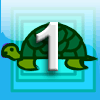First Forward Into Logo 1: Square Five

Age 7 to 16 Challenge Level:

A Short introduction to using Logo. This is the first in a twelve part series.